### Structural Equation Modeling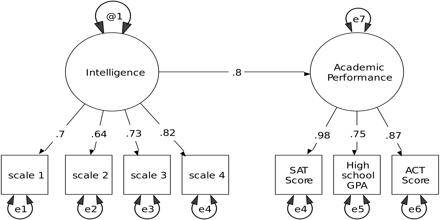Structural Equation Modeling (SEM) is a largely confirmatory, rather than exploratory, technique. It refers to a diverse set of mathematical models, computer algorithms, and statistical methods that fit networks of constructs to data. It is also is a very general, chiefly linear, chiefly cross-se.....

Read More »

### Path Analysis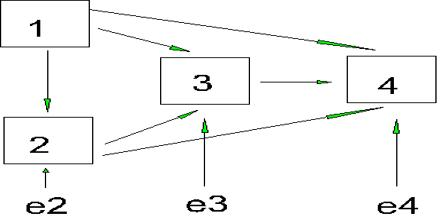This article describe about Path Analysis, which is developed as a method of decomposing correlations into different pieces for interpretation of effects. It is closely related to multiple regression. Path Analysis can be viewed as a special case of Structural Equation Modeling (SEM) – one in w.....

Read More »

### Mixture Model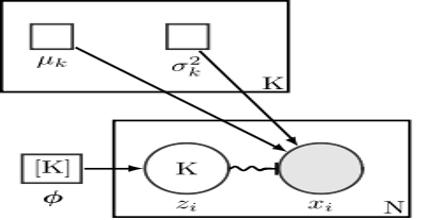Mixture Model is a type of probabilistic model which are capable of detecting similar groups of data. It is the process of grouping similar data is known as clustering, segmentation or density estimation. Mixture Model use to make statistical inferences about the properties of the sub-populations.....

Read More »

### Mixture Distribution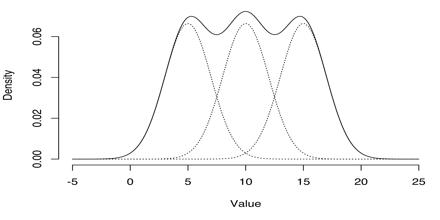This article talks about Mixture Distribution, which is arise in many contexts in the literature and arise naturally where a statistical population contains two or more subpopulations. It is discussed under the title of mixture models, while the present article concentrates on simple probabilisti.....

Read More »

### Graphical Model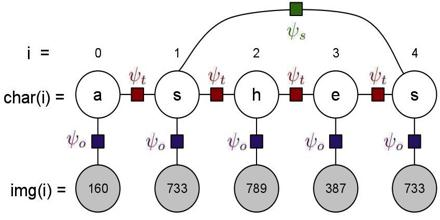This article focus on Graphical Model, which provide a general methodology for approaching bioinformatics, information retrieval, speech processing, image processing and communications problems. It is agnostic to the distinction between frequentist and Bayesian statistics. Generally, probabilist.....

Read More »

### Factor Graph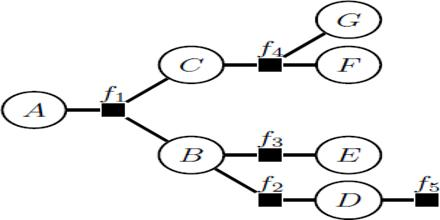Factor Graph is a type of probabilistic graphical model. It also used to represent factorization of a probability distribution function, enabling efficient computations, such as the computation of marginal distributions through the sum-product algorithm. Factor Graphs are compatible both with “.....

Read More »

### Chow Liu Tree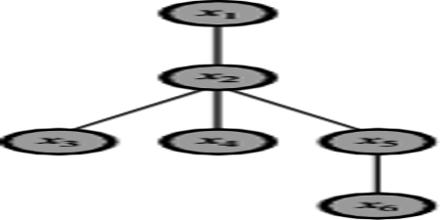Chow Liu Tree is capture both dependencies across time and dependencies across variables. Chow Liu Tree structures in the context of providing improved, yet tractable, models to address these problems in capturing output dependencies for HMMs. This article actually focus on Chow Liu Tree, which .....

Read More »

### Bayesian Inference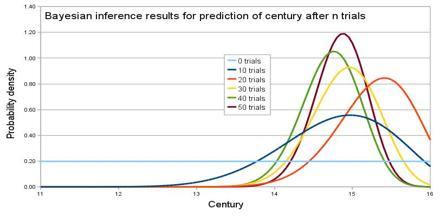Bayesian Inference is the process of updating probabilities of outcomes based upon the relationships in the model and the evidence known about the situation at hand.It is actually used, the end user applies evidence about recent events or observations. This information is applied to the model by .....

Read More »

### Multi-Label Classification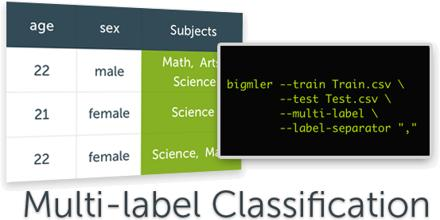Multi-Label Classification is the definition of concepts for the quantification of the multi-label nature of a data set. It is a binary relevance, which simply trains a classifier for each label independently. Multi-Label Classification should not be confused with multiclass classification, which.....

Read More »

### Prosecutor’s FallacyProsecutor’s Fallacy is any of several fallacies of statistical reasoning often used in legal arguments. It can arise from multiple testing, such as when evidence is compared against a large database. The size of the database elevates the likelihood of finding a match by pure chance alone; .....

Read More »

### Receiver Operating Characteristic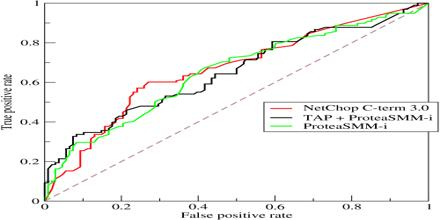This article talks about Receiver Operating Characteristic, which is a graphical plot that illustrates the performance of a binary classifier system as its discrimination threshold is varied. It generated by plotting the cumulative distribution function of the detection probability in the y-axis .....

Read More »

### Frequentist Probability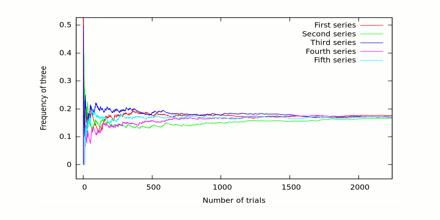Frequentist Probability concepts were introduced and much of probability mathematics derived, classical statistical inference methods were developed, the mathematical foundations of probability were solidified and current terminology was introduced. This article describe about Frequentist Probabi.....

Read More »